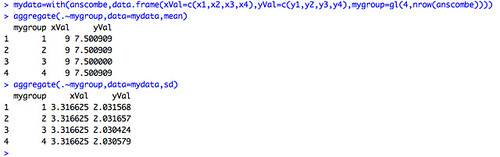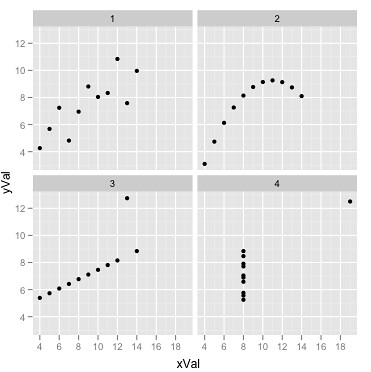Want to share your content on R-bloggers? click here if you have a blog, or here if you don't.

I spent a chunk of today trying to get my thoughts in order for a keynote presentation at next week’s The Difference that Makes a Difference conference. The theme of my talk will be on how visualisations can be used to discover structure and pattern in data, and as in many or my other recent talks I found the idea of Anscombe’s quartet once again providing a quick way in to the idea that sometimes the visual dimension can reveal a story that simple numerical analysis appears to deny.

For those of you who haven’t come across Anscombe’s quartet yet, it’s a set of four simple 2 dimensional data sets (each 11 rows long) that have similar statistical properties, but different stories to tell…

Quite by chance, I also happened upon a short exercise based on using R to calculate some statistical properties of the quartet (More useless statistics), so I thought I’d try and flail around in my unprincipled hack-it-and-see approach to learning R to see if I could do something similar with rather simpler primitives than described in that blog post.

(If you’re new to R and want to play along, I recommend RStudio…)

Here’s the original data set – you can see it in R simply by typing anscombe:

```   x1 x2 x3 x4    y1   y2    y3    y4
1  10 10 10  8  8.04 9.14  7.46  6.58
2   8  8  8  8  6.95 8.14  6.77  5.76
3  13 13 13  8  7.58 8.74 12.74  7.71
4   9  9  9  8  8.81 8.77  7.11  8.84
5  11 11 11  8  8.33 9.26  7.81  8.47
6  14 14 14  8  9.96 8.10  8.84  7.04
7   6  6  6  8  7.24 6.13  6.08  5.25
8   4  4  4 19  4.26 3.10  5.39 12.50
9  12 12 12  8 10.84 9.13  8.15  5.56
10  7  7  7  8  4.82 7.26  6.42  7.91
11  5  5  5  8  5.68 4.74  5.73  6.89```

We can construct a simple data frame containing just the values of x1 and y1 with a construction of the form: data.frame(x=c(anscombe\$x1),y=c(anscombe\$y1)) (where we identify the columns explicitly by column name) or alternatively data.frame(x=c(anscombe),y=c(anscombe)) (where we refer to them by column index number).

x y
1 10 8.04
2 8 6.95
3 13 7.58
4 9 8.81
5 11 8.33
6 14 9.96
7 6 7.24
8 4 4.26
9 12 10.84
10 7 4.82
11 5 5.68

A tidier way of writing this is as follows:
with(anscombe,data.frame(x1Val=c(x1),y1Val=c(y1)))

In order to call on, or refer to, the data frame, we assign it to a variable: g1data=with(anscombe,data.frame(xVal=c(x1),yVal=c(y1)))

We can then inspect the mean and sd values: mean(g1data\$xVal), or sd(g1data\$yVal)

> mean(g1data\$xVal)
 9
> sd(g1data\$xVal)
 3.316625
>

To plot the data, we can simply issue a plot command: plot(g1data)It would be possible to create similar datasets for each of the separate groups of data, but R has all sorts of tricks for working with data (apparently…?!;-) There are probably much better ways of getting hold of the statistics in a more direct way, but here’s the approach I took. Firstly, we need to reshape the data a little. I cribbed the “useless stats” approach for most of this. The aim is to produce a data set with 44 rows, and 3 columns: x, y and a column that identifies the group of results (let’s call them myX, myY and myGroup for clarity). The myGroup values will range from 1 to 4, identifying each of the four datasets in turn (so the first 11 rows will be for x1, y1 and will have myGroup value 1; then the values for x2, y2 and myGroup equal to 2, and so on). That is, we want a dataset that starts:

```1 10 9.14 1
2 8 8.14 1```

and ends:

```43 8 7.91 4
44 8 6.89 4```

To begin with, we need some helper routines:

– how many rows are there in the data set? nrow(anscombe)
– how do we create a set of values to label the rows by group number (i.e. how do we generate a set of 11 1′s, then 11 2′s, 11 3′s and 11 4′s)? Here’s how: gl(4, nrow(anscombe)) [typing ?gl in R should bring up the appropriate help page;-) What we do is construct a list of 4 values, with each value repeating nrow(anscombe) times]
– to add in a myGroup column to a dataframe containing x1 and y1 columns, set with just values 1, we simply insert an additional column definition: data.frame(xVal=c(anscombe\$x1), yVal=c(anscombe\$y1), mygroup=gl(1,nrow(anscombe)))
– to generate a data frame containing three columns and the data for group 1, followed by group 2, we would use a construction of the form: data.frame(xVal=c(anscombe\$x1,anscombe\$x2), yVal=c(anscombe\$y1,anscombe\$y2), mygroup=gl(2,nrow(anscombe))). That is, populate the xVal column with rows from x1 in the anscombe dataset, then the rows from column x2; populate yVal with values from y1 then y2; and populate myGroup with 11 1′s followed by 11 2′s.
– a more compact way of constructing the data frame is to specify that we want to concatenate (c()) values from several columns from the same dataset: with(anscombe,data.frame(xVal=c(x1,x2,x3,x4), yVal=c(y1,y2,y3,y4), mygroup=gl(4,nrow(anscombe))))
– to be able to reference this dataset, we need to assign it to a variable: mydata=with(anscombe,data.frame(xVal=c(x1,x2,x3,x4), yVal=c(y1,y2,y3,y4), mygroup=gl(4,nrow(anscombe))))

This final command will give us a data frame with the 3 columns, as required, containing values from group 1, then group 2, then groups 3 and 4, all labelled appropriately.

To find the means for each column by group, we can use the aggregate command: aggregate(.~mygroup,data=mydata,mean)

(I think you read that as follows:”aggregate the current data (.) by each of the groups in (~) mygroup using the mydata dataset, reporting on the groupwise application of the function: mean)

To find the SD values: aggregate(.~mygroup,data=mydata,sd)Cribbing an approach I discovered from the hosted version of the ggplot R graphics library, here’s a way of plotting the data for each of the four groups from within the single aggregate dataset. (If you are new to R, you will need to download and install the ggplot2 package; in RStudio, from the Packages menu, select Install Packages and enter ggplot2 to download and install the package. To load the package into your current R session, tick the box next to the installed package name or enter the command library("ggplot2").)

The single command to plot xy scatterplots for each of the four groups in the combined 3 column dataset is as follows:

ggplot(mydata,aes(x=xVal,y=yVal,group=mygroup))+geom_point()+facet_wrap(~mygroup)

And here’s the result (remember, the statistical properties were the same…)To recap the R commands:

mydata=with(anscombe,data.frame(xVal=c(x1,x2,x3,x4), yVal=c(y1,y2,y3,y4), group=gl(4,nrow(anscombe))))
aggregate(.~mygroup,data=mydata,mean)
aggregate(.~mygroup,data=mydata,sd)
library(ggplot2)
ggplot(mydata,aes(x=xVal, y=yVal)) + geom_point() + facet_wrap(~mygroup)

PS this looks exciting from an educational and opendata perspective, though I haven’t had a chance to play with it: OpenCPU: a server where you can upload and run R functions. (The other hosted R solutions I was aware of – R-Node – doesn’t seem to be working any more? online R-Server [broken?]. For completeness, here’s the link to the hosted ggplot IDE referred to in the post. And finally – if you need to crucnh big files, CloudNumbers may be appropriate (disclaimer: I haven’t tried it))

PPS And here’s something else for the data junkies – an easy way of getting data into R from Datamarket.com: How to access 100M time series in R in under 60 seconds.